The mole exercises

Be =9, K =39, Cl =35.5, Ca =40,O =16, C =12, H =1, Na =23
S =32.

 Compound Mass Mole Number of units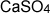3 mole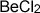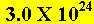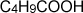40 grams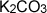6 mole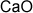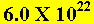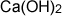5.6 grams0.136 grams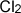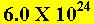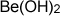4 mole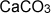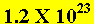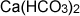0.3 mole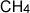0.16 Kilograms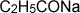0.5 mole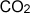100 mole